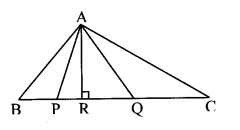# Maharashtra Board Class 10 Maths Solutions Chapter 1 Similarity Practice Set 1.1

## Maharashtra State Board Class 10 Maths Solutions Chapter 1 Similarity Practice Set 1.1

Question 1.
Base of a triangle is 9 and height is 5. Base of another triangle is 10 and height is 6. Find the ratio of areas of these triangles.
Solution:
Let the base, height and area of the first triangle be b1, h1, and A1 respectively.
Let the base, height and area of the second triangle be b2, h2 and A2 respectively.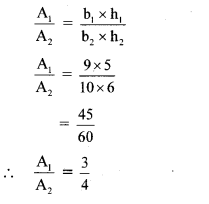[Since Ratio of areas of two triangles is equal to the ratio of the product of their bases and corresponding heights]
∴ The ratio of areas of the triangles is 3:4.

Question 2.
In the adjoining figure, BC ± AB, AD _L AB, BC = 4, AD = 8, then find $$\frac{A(\Delta A B C)}{A(\Delta A D B)}$$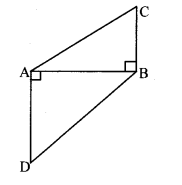Solution:
∆ABC and ∆ADB have same base AB.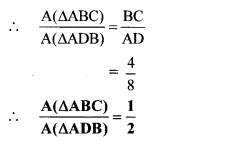[Since Triangles having equal base]

Question 3.
In the adjoining figure, seg PS ± seg RQ, seg QT ± seg PR. If RQ = 6, PS = 6 and PR = 12, then find QT.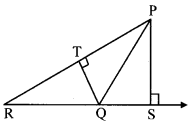Solution:
In ∆PQR, PR is the base and QT is the corresponding height.
Also, RQ is the base and PS is the corresponding height.
$$\frac{A(\Delta P Q R)}{A(\Delta P Q R)}=\frac{P R \times Q T}{R Q \times P S}$$ [Ratio of areas of two triangles is equal to the ratio of the product of their bases and corresponding heights]
∴ $$\frac{1}{1}=\frac{P R \times Q T}{R Q \times P S}$$
∴ PR × QT = RQ × PS
∴ 12 × QT = 6 × 6
∴ QT = $$\frac { 36 }{ 12 }$$
∴ QT = 3 units

Question 4.
In the adjoining figure, AP ⊥ BC, AD || BC, then find A(∆ABC) : A(∆BCD).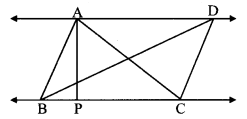Solution:
Draw DQ ⊥ BC, B-C-Q.∴ AP = DQ   (i)  [Perpendicular distance between two parallel lines is the same]
∆ABC and ∆BCD have same base BC.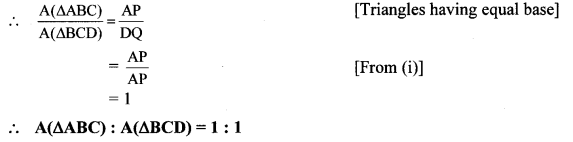Question 5.
In the adjoining figure, PQ ⊥ BC, AD ⊥ BC, then find following ratios.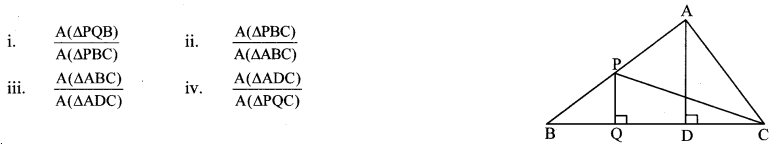Solution:
i. ∆PQB and tPBC have same height PQ.ii. ∆PBC and ∆ABC have same base BC.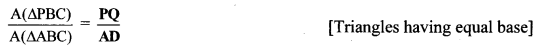Find $$\frac{A(\Delta A B C)}{A(\Delta A P Q)}$$